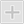Viewed products

NewView larger

# BRASS/RUBBER BEARING

New product

Water-lubricated rubber bearing. Brass shell-rubber lined cutless bearing specifically designed for water lubricated sterntubes and rudder stocks.

31,54 € tax incl.230131ANCO1 = 25,401 1/2" = 38,104" = 101,60 31,54 € IVA incl.230132AGRA1 = 25,401 1/4" = 31,754" = 101,60 67,60 € IVA incl.230133AOST1 1/8 = 28,571 5/8" = 41,274 1/2" = 114,30 46,74 € IVA incl.230134ARGE1 1/4 = 31,751 3/4" = 44,455" = 127 58,53 € IVA incl.230135BERG1 3/8 = 34,921 7/8" = 47,625 1/2" = 139,70 66,05 € IVA incl.230136BRES1 1/2 = 38,102" = 50,806" = 152,40 73,06 € IVA incl.230137BULL1 5/8 = 41,272 1/8" = 53,976 1/2" = 165,10 103,34 € IVA incl.230139BARI1 7/8 = 47,622 5/8" = 66,677 1/2" = 190,50 251,60 € IVA incl.230141COMO2 1/8 = 53,973" = 76,208 1/2" = 215,90 308,74 € IVA incl.230142CHIA2 1/4 = 57,153" = 76,209" = 228,60 141,70 € IVA incl.230143CAMO2 3/8 = 60,323 1/4" = 82,559 1/2" = 241,30 162,92 € IVA incl.230144CALT2 1/2 = 63,503 1/4" = 82,5510" = 254 187,11 € IVA incl.230145CADO2 5/8 = 66,673 3/8" = 85,7210 1/2" = 266,70 249,00 € IVA incl.230146CANT2 3/4 = 69,853 3/4" = 95,2511" = 279,40 317,53 € IVA incl.230147CAMA3 3/8 = 85,724 1/2" = 114,3014" = 355,60 825,09 € IVA incl.230150-1 3/4 = 44,452 5/8" = 66,677" = 177,80 141,52 € IVA incl.230151CANI3 1/2 = 88,904 1/2" = 114,3014" = 355,60 430,59 € IVA incl.230152-2 1/4 = 57,153 1/8" = 79.389" = 228,60 274,38 € IVA incl.230155-1 1/4 = 31,751 1/2" = 38,105" = 127 55,21 € IVA incl.230156-1 1/2 = 38,101 7/8" = 47,626" = 152,40 152,01 € IVA incl.230157-2 1/2 = 63,503 1/8" = 79,3710" = 254 394,03 € IVA incl.230179-4 = 101.605 1/4" = 133.3516" = 406.40 133,75 € IVA incl.230193BLEV1 3/4 = 44,452 3/8" = 60,327" = 177,80 90,82 € IVA incl.230194BOLO2 = 50,802 5/8" = 66,678" = 203,20 110,07 € IVA incl.